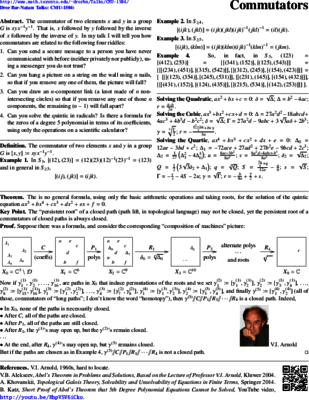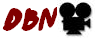© | << < ? > >> | Dror Bar-Natan: Talks:

# Two Talks at Carnegie Mellon University, April 3, 2015## Commutators

Abstract. The commutator of two elements $x$ and $y$ in a group $G$ is $xyx^{-1}y^{-1}$. That is, $x$ followed by $y$ followed by the inverse of $x$ followed by the inverse of $y$. In my talk I will tell you how commutators are related to the following four riddles:

1. Can you send a secure message to a person you have never communicated with before (neither privately nor publicly), using a messenger you do not trust?
2. Can you hang a picture on a string on the wall using $n$ nails, so that if you remove any one of them, the picture will fall?
3. Can you draw an $n$-component link (a knot made of $n$ non-intersecting circles) so that if you remove any one of those $n$ components, the remaining $n-1$ will fall apart?
4. Can you solve the quintic in radicals? Is there a formula for the zeros of a degree $5$ polynomial in terms of its coefficients, using only the operations on a scientific calculator?

Prerequisites.

1. The first week of any class on group theory.
2. Knowing that every complex number other than to $0$ has exactly $n$ roots of order $n$, and how to compute them.

Handout: Commutators-Handout.pdf. Slides: Commutators-Slides.nb. Talk video:. Sources: pensieve.

## The Kashiwara-Vergne Problem and Topology

### Mathematics Colloquium

Abstract. I will describe a general machine, a close cousin of Taylor's theorem, whose inputs are topics in topology and whose outputs are problems in algebra. There are many inputs the machine can take, and many outputs it produces, but I will concentrate on just one input/output pair. When fed with a certain class of knotted 2-dimensional objects in 4-dimensional space, it outputs the Kashiwara-Vergne Problem (1978, solved Alekseev-Meinrenken 2006, elucidated Alekseev-Torossian 2008-2011), a problem about convolutions on Lie groups and Lie algebras.

A repeat of my November 2013 Bern Colloquium, see video there.

Handout: KVT.html, KVT.pdf, KVT.png.
See the papers: WKO1, WKO2.

Sources: pensieve.